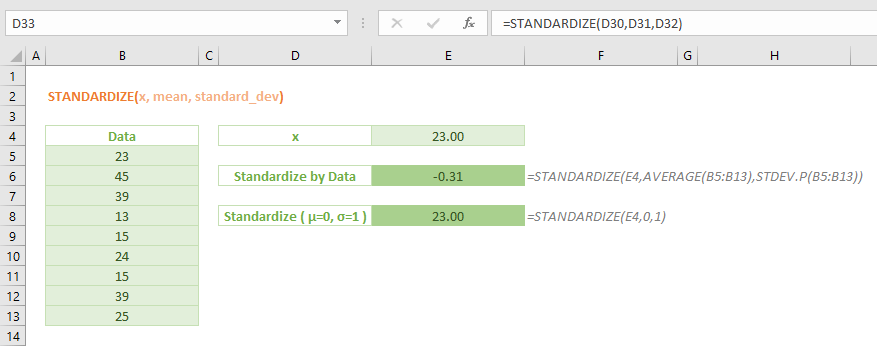The STANDARDIZE Excel function is a Statistical formla that calculates and returns normalized value (z-score) based on the mean and standard deviation of a data set. In this guide, we’re going to show you how to use the STANDARDIZE function and also go over some tips and error handling methods.

## Supported versions

• All Excel versions

## STANDARDIZE Excel Function Syntax

STANDARDIZE(x, mean, standard_dev)

## Arguments

 x The numeric value you want to normalize mean The arithmetic mean (average) of the distribution standard_dev The standard deviation of the distribution

## STANDARDIZE Function Examples

### If you have the mean and standard deviation

If you have the arithmetic mean and standard deviation values of the distribution, use them with the value you want to normalize (x).

=STANDARDIZE(x, mean, standard deviation)### If you don’t have the mean and standard deviation

If you don’t have the arithmetic mean and standard deviation, you can easily calculate them from the data set. Use the following functions to calculate these values and use them in the STANDARDIZE function.

• You can use the AVERAGE function to find the arithmetic mean (average) of the dataset.
• You can use either the STDEV.P or the STDEV.S function to calculate the standard deviation of the entire population or a sample data correspondingly.### Calculating z-score for entire dataset

You can calculate the standard scores (z-score) for the entire data set by evaluating STANDARDIZE. Simply use each value as the x argument by using the arithmetic mean and standard deviation of the same data set. Remember to lock the references before copying the formula if the mean and standard deviation values are in their own respective cells.## Issues

### #NUM!

If standard_dev ≤ 0.